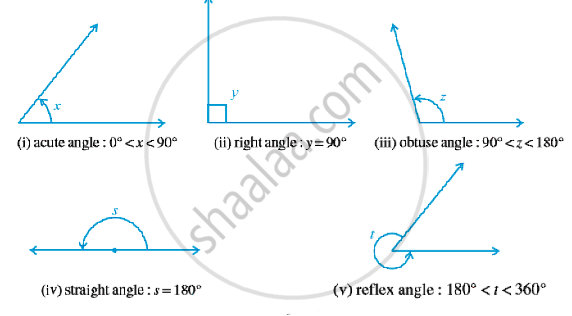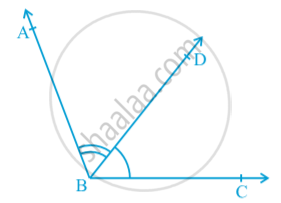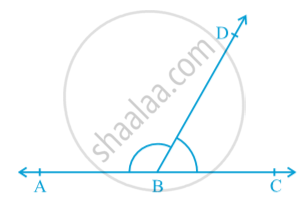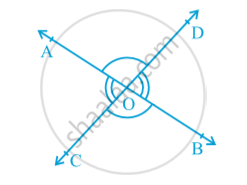# Basic Terms and Definitions

#### notes

A line with two end points is called a line-segment and a part of a line with one end point is called a ray.  Note that the line segment AB is denoted by bar (AB) . The ray AB is denoted by vec (AB) , and a linbe is denoted by AB. However, we will not use these symbols, and will denote the line segment AB, ray AB, length AB and line AB by the same symbol, AB.
If three or more points lie on the same line, they are called collinear points; otherwise they are called non-collinear points.
An angle is formed when two rays originate from the same end point. The rays making an angle are called the arms of the angle and the end point is called the vertex of the angle. There are different types of angles , such as acute angle, right angle, obtuse angle, straight angle and reflex angle in following fig.Further, two angles whose sum is 90° are called complementary angles, and two angles whose sum is 180° are called supplementary angles.
Two angles are adjacent, if they have a common vertex, a common arm and their non-common arms are on different sides of the common arm. In following fig.∠ ABD and ∠ DBC are adjacent angles. Ray BD is their common arm and point B is their common vertex. Ray BA and ray BC are non common arms.  Moreover, when two angles are adjacent, then their sum is always equal to the angle formed by the two noncommon arms. So , ∠ ABC = ∠ ABD + ∠ DBC .
Note that ∠ ABC and ∠ ABD are not adjacent angles.  Because their noncommon arms BD and BC lie on the same side of the common arm BA.
If the non-common arms BA and BC in following fig .form a line then it will look like in above figure. In this case,
∠ ABD and ∠ DBC are called linear pair of angles.
The vertically opposite angles formed when two lines, say AB and CD, intersect each other, say at the point O in following fig.There are two pairs of vertically opposite angles. One pair is ∠AOD and ∠BOC.

If you would like to contribute notes or other learning material, please submit them using the button below.

### Shaalaa.com

Basic Terms and Definitions [00:14:27]
S
0%